# integral

Also found in: Dictionary, Thesaurus, Medical, Acronyms, Wikipedia.
Related to integral: integral calculus, Integral part

## integral

1. Maths
a. of or involving an integral
b. involving or being an integer
2. Maths the limit of an increasingly large number of increasingly smaller quantities, related to the function that is being integrated (the integrand). The independent variables may be confined within certain limits (definite integral) or in the absence of limits (indefinite integral).
Collins Discovery Encyclopedia, 1st edition © HarperCollins Publishers 2005

## INTEGRAL

(in -tĕ-grăl, in-teg -răl) (International Gamma-Ray Astrophysics Laboratory) A European Space Agency (ESA) space observatory, launched Oct. 2002 aboard a Proton rocket from Baikonur in Kazakhstan and comprising four high-energy astronomical telescopes. The 4-tonne satellite, developed and operated by ESA in conjunction with Russia and the USA, was built to detect some of the most energetic radiation emanating from space, including that from gamma-ray bursts and neutron stars. Its achievements include spectral measurements of numerous gamma-ray sources and the mapping of the galactic plane in gamma radiation, the detection of some of the faintest and closest gamma-ray bursts ever found, the resolution of diffuse gamma-ray emissions from the center of the Milky Way Galaxy, the increase of our knowledge of active galactic nuclei, and the discovery of a new class of highly absorbed object. In a mission intended to last for two years, extendable to three, INTEGRAL followed a highly eccentric orbit (period 72 hours, inclination 51.6°) that carried it from 9000 km to 153 000 km above the Earth. Its four science instruments were (1) IBIS, a gamma-ray imager operating over the range 50–100 000 keV and imaging the sky at the arcminute level; (2) SPI, a gamma-ray spectrometer with a functional spectral range of 15–10 000 keV and a spectral resolution of about 500; (3) JEM-X, an X-ray monitor operating over the range 4–100 keV; and (4) OMC, an optical monitor with CCD and lens optics designed to detect the afterglow from a gamma-ray burst and identify the object associated with it.
Collins Dictionary of Astronomy © Market House Books Ltd, 2006
The following article is from The Great Soviet Encyclopedia (1979). It might be outdated or ideologically biased.

## Integral

one of the most important concepts of mathematics, answering the need to find functions given their derivatives (for example, to find the function expressing the path traversed by a moving point given the velocity of that point), on the one hand, and to measure areas, volumes, lengths of arcs, the work done by forces in a given interval of time, and so forth, on the other. These problems involve so-called indefinite integrals and definite integrals. Their computation is the task of the integral calculus.

Indefinite integral. An antiderivative of a function f (x) of a single real variable is a function F (x) whose derivative for each value of (x) is equal to f(x). By adding a constant to an antiderivative of a function, we again obtain an antiderivative of that function. Consequently, having one antiderivative F (x) of a function f (x), we obtain a general expression for all antiderivatives of that function in the form F (x) + C. This general expression for antiderivatives is called the indefinite integral

∫f(x) dx

of the function f(x). One of the fundamental theorems of the integral calculus establishes the fact that every continuous function f(x) of a real variable has an indefinite integral.

Definite integral. The definite integral of a function f(x) with lower limit a and upper limit b may be defined as the difference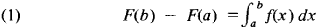where F(x) is an antiderivative of the function f(x). This definition does not depend on which antiderivative is chosen for the computation of the definite integral. If the function f(x) is continuous, the above definition is equivalent for the case a < b to the following definition given by A. Cauchy (1823): Consider an arbitrary partition of the interval [a, b] determined by the points

(2) a = x0 < X1 < … < xn = b

In each subinterval [x11,Xi] (i = 1, 2, …, n) we take an arbitrary point ξ i (i 1 ≤ ξ ixi) and form the sum

(3) Sn = f1)(x1-x0)+f2)(x2-x1)+ … + fn)(xn-xn_1)

The sum Sn depends on the choice of the points xi, and ξi. However, in the case of a continuous function f(x), the sums Sn obtained by choosing different points xi and ξi, converge to the same limit provided that the maximum of the differences xi— Xi, 1 tends to zero as n—∞. This limit is precisely the definite integral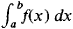We define: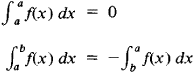As noted above, the definite integral can be expressed by means of any antiderivative F(x). Conversely, an antiderivative F(x) can be written in the form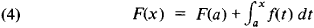where a is an arbitrary constant. Accordingly, the indefinite integral can be written in the form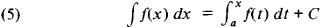For the evolution of the concept of the integral and the properties of indefinite and definite integrals seeINTEGRAL CALCULUS.

Generalization of the concept of the integral.

Riemann integral Cauchy applied his definition of the integral only to continuous functions. B. Riemann (1853) defined the integral.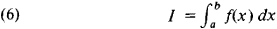as the limit of the sums Sn as max(xi – xi_1)→ 0 in all those cases when this limit is uniquely defined. He also investigated the conditions of applicability of such a definition. H. Lebesgue gave (1902) a more complete form to these conditions using his concept of the measure of a set. Two conditions are necessary and sufficient for a function f(x) to be integrable over [a, b] in the sense of Riemann: f(x) must be bounded on [a,b], and the set of discontinuities of the function f(x) on [a,b] must have measure zero. Thus, continuity at each point of the interval [a,b] is not at all required for Riemann integrability.

It is now possible to define the antiderivative and the indefinite integral by means of formulas (4) and (5). However, it should be noted that in this case the antiderivative F(x) does not necessarily have the integrand f(x) as its derivative at every point. But at every point where f(x) is continuous, that is, by virtue of Lebesgue’s result, everywhere except perhaps on a set of measure zero, we have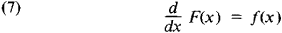G. Darboux (1879) gave a definition of the Riemann integral rendering the conditions for the existence of such an integral intuitively clear. In place of the sums (3) Darboux introduced the sums (called Darboux sums)

S̅ = M1(x1–x0) + M2(x2 – x1)+…+Mn(xn–xn-1)

S = m1(xl – x0) + m2(x2 - x1) + … + mn(xn–xn-1)

where Mk is the least upper bound of the function f(x) on the subinterval [xk _ 1,xk] and mk is the greatest lower bound of f(x) on that subinterval. If I is the greatest lower bound of the sums and I is the least upper bound of the sums , then the condition ̲ I = Ī is necessary and sufficient for the existence of the Riemann integral. The common value I = Ī = of the numbers and Ī is the Riemann integral (6). The numbers Ī and I are called, respectively, the upper and lower Darboux integrals.

Lebesgue integral. The concept of the measure of sets introduced by Lebesgue permitted a significantly broader definition of the integral. In order to define the integral (6), Lebesgue divides the range of possible values of the variable y = f(x) by means of points

… < y-2 < y-i < y0 < … < yi < …

and designates as Mi the set of those points x in the interval [a, b] for which

yi-1f(x) < yi

The sum S is defined by the equality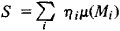where ηi is selected from the interval yi–1 ≤ ηi, < yi, and μ (Mi) denotes the measure of Mi. The function f(x) is said to be integrable in the sense of Lebesgue on the interval [a,b] if the series that define the sums S converge absolutely as max(yi, – yi1) → 0. The limit of these sums is called the Lebesgue integral (6). One may define the antiderivative in the sense of Lebesgue as a function F(x) satisfying the equality (4), where the integral on the right is understood to be the Lebesgue integral. As in the case of the Riemann integral, equality (7) will in this case be fulfilled at all points except, perhaps, on a set of measure zero.

For a bounded function f(x) to be Lebesgue integrable it is necessary and sufficient that it belong to the class of functions measurable in the sense of Lebesgue. All functions that are encountered in mathematical analysis are measurable in this sense. Moreover, up to the present (1972) not a single specific example of a nonmeasurable function has been constructed. Thus, for the case of bounded functions, Lebesgue has solved the problem of defining the integral (6) with a generality that exhausts the requirements of mathematical analysis. Among Lebesgue integrable functions, there are infinitely many functions that are everywhere discontinuous and, consequently, not Riemann integrable. However, every Riemann integrable function is also Lebesgue integrable.

Lebesgue’s definition can be generalized to include integration over a half-line or the whole line, that is, to the case of integrals of the form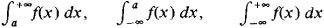After this generalization, Lebesgue’s theory encompasses all cases of absolutely convergent improper integrals.

The generality attained in Lebesgue’s definition is essential for many problems of mathematical analysis; for example, only after the introduction of the Lebesgue integral was it possible to establish the Riesz-Fischer theorem in the theory of trigonometric series, which asserts that any series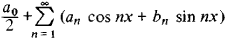for which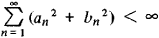represents the function f(x), for which the coefficients an and bn have the values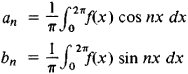(the integrals are Lebesgue integrals).

Stieltjes integral. At the end of the 19th century, the Riemann integral was generalized in a manner that differed completely from that resulting from the introduction of the concept of Lebesgue measure. This generalization was due to T. Stieltjes (1894). Specifically, let f(x) be a continuous function of a real variable x defined on the interval [a, b], and let U(x) be a bounded monotonic (nondecreasing or nonincreasing) function defined on the same interval. For the definition of the Stieltjes integral we take an arbitrary partition (2) of the interval [a,b] and form the sum

f1) [U(x1) – U(x0) + f2)[U(x2)– U(x1)] + … + f(ξn)[U(xn) – (U(xn-1)]

where ξ1, ξ2, …, ξn are arbitrary points chosen from the subintervals [x0, x1], [xi, x2], …, [xn – 1, xn], respectively. Let δ be the largest distance between two neighboring points of the partition (2). If we take any sequence of partitions for which δ tends to zero, then the sum (8) tends to be the same limit no matter which points ξ1, ξ2, …, are selected in the respective intervals. This limit is termed, following Stieltjes, the integral of the function f(x) with respect to the function U(x) and is denoted by the symbol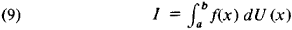The integral (9) (also called the Stieltjes integral) exists even in the case when the bounded function U(x), while not itself mono-tonic, can be represented in the form of a sum or difference of two bounded monotonic functions U1(x) and U2(x):

U(x) = U1(x)– U2(x)

that is, when it is a function of bounded variation.

If the integrator U(x) has a bounded and Riemann integrable derivative U’(x), then the Stieltjes integral is related to the Riemann integral, namely,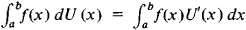In particular, when U(x) = x + C, the Stieltjes integral (9) becomes the ordinary Riemann integral (6).

Further generalizations. The concepts of the integral owing to Stieltjes and Lebesgue were subsequently successfully combined and generalized to yield a concept of integration over any (measurable) set in a space of any number of dimensions. Classical multiple integrals are fully covered by this approach. The requirements of disciplines such as probability theory and the general theory of dynamic systems have led to the still broader concept of the abstract Lebesgue integral, which is based on the general concepts of measure of sets and measurability of functions. Specifically, let X be a space with a system B of its subsets called measurable. It is assumed that B is closed under the usual set-theoretical operations performed finitely many or countably many times. Let μ, be a finite measure on B. For a 5-measurable function y = f(x), xX, assuming a finite or countable number of values y1, y2, …, yn, …, respectively, on the pairwise disjoint sets A1, …, An, …, whose sum is X, we define the integral of the function f(x) with respect to the measure μ, to be the sum of the series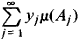with the assumption that this series converges absolutely. The integral just defined is denoted by the symbol

xf(x)μ(dx)

For other f integrability and the integral are defined by a certain natural passage to the limit involving the “piecewise constant” functions introduced above.

Let A be a measurable set and let ϕ A(x) = 1 for x belonging to A and ϕ A(x) = 0 for x not belonging to A. Then the integral of f(x) on the set A is defined by

Af(x)μ (dx) = ∫ xf(x)ϕA(x)μ(dx)

When μ and A are fixed, the integral, viewed as a function of f, may be regarded as a linear functional; when f is fixed, the integral, viewed as a function of the set A, is a countably additive function.

It should be noted that despite its apparent abstractness this general concept of an integral is very well suited for the definition of such a concept as mathematical expectancy (in probability theory) and even for the general formulation of the testing of statistical hypotheses. Integrals with respect to the so-called Wiener measure and its various analogs are used in statistical physics (here X is the space of functions continuous on some interval). The generalizations of the concept of the integral mentioned so far have been such that f and f prove to be either both integrable or both nonintegrable.

Generalizations of the initial concept of the integral in another direction apply to functions of a single variable, but they contribute much more to the study of the integration of unbounded functions. Already Cauchy, in the case of a function f(x) unbounded at a point x = c, defined the integral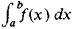when a < c < b, as the limit of the expression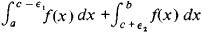as ξ1 → 0 and ξ2 → 0. Analogously, the integral with infinite limits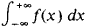is defined as the limit of the integral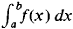as a → – ∞ and b → + ∞. If in this case the integrability of f(x) is not required, then this definition of Cauchy is not covered by Lebesgue’s definition.

A still broader generalization of the concept of the integral in this direction was proposed by A. Denjoy (1912) and A. Ia. Khinchin (1915).

### REFERENCES

Lebesgue, H. Integrirovanie i otyskanie primitivnykh funktsii. Moscow-Leningrad, 1934. (Translated from French.)
Saks, S. Teoriia integrala. Moscow, 1949. (Translated from English.)
Kamke, E. Integral Lebega-Stilt’esa. Moscow, 1959. (Translated from German.)
Whitney, H. Geometricheskaia teoriia integrirovaniia. Moscow, 1966. (Translated from English.)
Rudin, W. Osnovy matematicheskogo analiza. Moscow, 1962. (Translated from English.)
Dunford, N., and J. T. Schwarz. Lineinye operatory: Obschaia teoriia. Moscow, 1962. (Translated from English.)
Neveau, J. Matematicheskie osnovy teorii veroiatnostei. Moscow, 1969. (Translated from French.)
Federer, H. Geometric Measure Theory. Berlin-Heidelberg-New York, 1969.

A. N. KOLMOGOROV

## integral

[′int·ə·grəl]
(mathematics)
A solution of a differential equation is sometimes called an integral of the equation.
An element a of a ring B is said to be integral over a ring A contained in B if it is the root of a polynomial with coefficients in A and with leading coefficient 1.
McGraw-Hill Dictionary of Scientific & Technical Terms, 6E, Copyright © 2003 by The McGraw-Hill Companies, Inc.
References in periodicals archive ?
If the lower limit of integration is constant and the upper one is variable we are in the case of Volterra integral equations which is our subject.
developed in  the fuzzy Laplace transform method to solve fuzzy convolution Volterra integral equation (FCVIE) of the second kind.
The first integral, shown in (8) or (9), maybe quite simple for practical problems if f(x) is an analytically integrable function.
For a function f : [a,b] [right arrow] R we denote the Lebesgue integral of f on a measurable E [subset] [a, b], when it exists, by (L) [[integra].sub.E] f.
As an illustration, we show how this method can be applied to solve the following integral :
Memou, "Boundary value problem with integral conditions for a linear third-order equation," Journal of Applied Mathematics, vol.
The above integral can be calculated taking x = 0 in (3); thus,
The series accuracy [DELTA][f.sup.[alpha]] = [f.sup.[alpha].sub.N] - [f.sup.[alpha].sub.L] is shown in Figure 1 where the quantities [f.sup.[alpha].sub.L] are the values of integral for L < N = N' .As can be seen from Figure 1, for given [alpha], the convergence rates are satisfactory for arbitrary values of parameter t.
Over the next nine months, the combined Union Insurance and CSC teams will manage the implementation, customization, training and testing of the Integral components.
Integral BioSystems is experienced working with all types of drug development entities in the US and around the world.
In this paper weighted double integral Montgomery identity is proved and there are some new double integral q- Chebychev Gruss type inequalities are derived.
ESOTERIQUE and Integral go head-to-head for the fifth time in the Kingdom of Bahrain Sun Chariot Stakes and the first-named is expect to emerge victorious at Newmarket.

Site: Follow: Share:
Open / Close